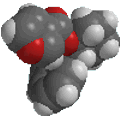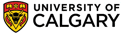﻿ DiolsBasic IUPAC Organic Nomenclature

### Diols (or polyols)

The term diol simply implies the presence of two alcohols. Polyols contain two or more -OH groups.
• The root name is based on the longest chain containing both the alcohol groups.
• The chain is numbered so as to give the one of the alcohol groups the lowest possible number (i.e. first point of difference).
• The appropriate multiplier (i.e. di- for two, tri for three etc.) is inserted before the -ol suffix or before the root.
 Functional group is an alcohol, therefore suffix = -ol Hydrocarbon structure is an alkane therefore -ane There are two alcohols, so insert the multiplier di The longest continuous chain is C2 therefore root = eth Locants for -OH units are 1- and 2- ethane-1,2-diol or 1,2-ethanediolHOCH2CH2OH Functional group is an alcohol, therefore suffix = -ol Hydrocarbon structure is an alkane therefore -ane There are two alcohols, so insert the multiplier di The longest continuous chain is C3 therefore root = prop Locants for -OH units are 1- and 2- propane-1,2-diol or 1,2-propanediolCH3CH(OH)CH2OH Functional group is an alcohol, therefore suffix = -ol Hydrocarbon structure is an alkane therefore -ane There are two alcohols, so insert the multiplier di The longest continuous chain is C4 therefore root = but Locants for -OH units are 1- and 4- butane-1,4-diol or 1,4-butanediolHOCH2CH2CH2CH2OH Functional group is an alcohol, therefore suffix = -ol Hydrocarbon structure is an alkane therefore -ane There are two alcohols, so insert the multiplier di The ring is C6 therefore root = cyclohex Locants for -OH units are 1- and 2- cyclohexane-1,2-diol or 1,2-cyclohexanediol©Dr. Ian Hunt, Department of Chemistry